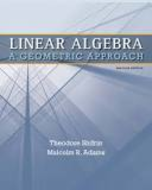### Solution Found!# Show (by calculation) that for any real numbers a and b, the matrices 1 a 0 2 _ and 1 b

Chapter 4, Problem 17

#### (choose chapter or problem)

Get Unlimited Answers! Check out our subscriptions

QUESTION:

Show (by calculation) that for any real numbers a and b, the matrices 1 a 0 2 _ and 1 b 0 2 _ are similar. (Hint: Remember that when P is invertible, B = P 1AP PB = AP.)

Step 1 of 3

LetandThen A and B are said to similar if there exists another matrix S such thatBecome a subscriber and get unlimited answers!